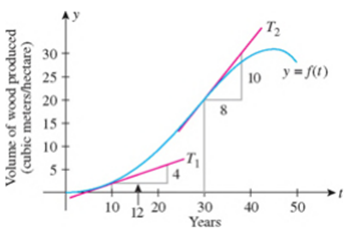Chapter 2.6, Problem 2E

Chapter
Section
Textbook Problem

FORESTRY The following graph shows the volume of wood produced in a single-species forest. Here, f(t) is measured in cubic meters per hectare, and t is measured in years. By computing the slopes of the respective tangent lines, estimate the rate at which the wood grown is changing at the beginning of year 10 and at the beginning of year 30.Source: The Random House Encyclopedia.To determine
The slopes of the tangent lines to estimate the rate of change in wood production at the beginning of year 10 and 30.

Explanation

Given:

The volume of wood produced in a single-species forest is shown in the given graph,

Figure (1)

Calculation:

From Figure (1), it is noticed that at the beginning of year 10 the curve has tangent line T1 . The value of rise for the line T1 is 4 and value of run is 12.

The slope of a straight line is the ratio of rise and run.

slope=riserun (1)

Substitute 4 for rise and 12 for run in the equation (1), to find the slope of the tangent at the beginning of year 10.

slope=412=13=0.33330.33

The slope of the tangent line at the beginning of year 10 is 0.33cubicmeters/hectare

Still sussing out bartleby?

Check out a sample textbook solution.

See a sample solution

The Solution to Your Study Problems

Bartleby provides explanations to thousands of textbook problems written by our experts, many with advanced degrees!

Get Started

Solve the equations in Exercises 126. x+4x+1+x+43x=0

Finite Mathematics and Applied Calculus (MindTap Course List)

In problems 23-58, perform the indicated operations and simplify. (x5+5x7)(x+1)

Mathematical Applications for the Management, Life, and Social Sciences

Evaluate the integral. 26. 0/4sec6tan6d

Single Variable Calculus: Early Transcendentals